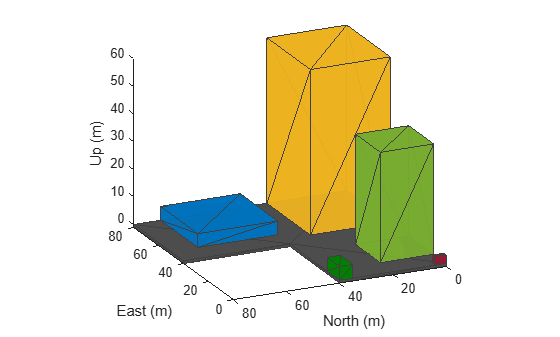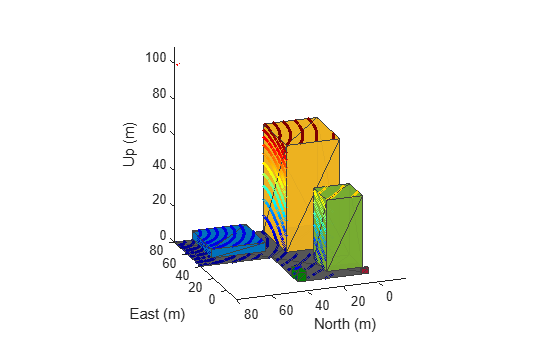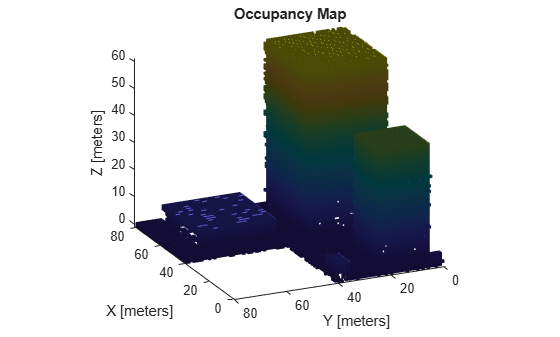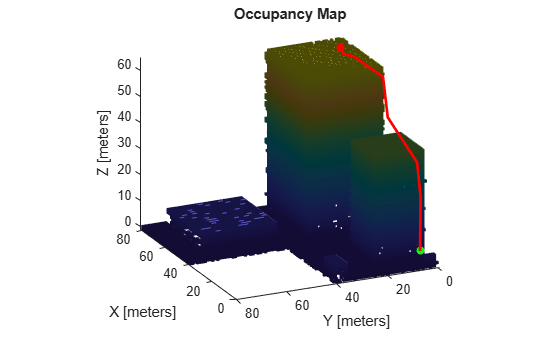# Map Environment For Motion Planning Using UAV Lidar

This example demonstrates how to use a UAV, equipped with a lidar sensor, to map an environment in a 3D occupancy map. That generated occupancy map can then be used to execute motion planning. The UAV follows a specified trajectory through the environment. Knowledge of the position of the UAV and the point clouds obtained at corresponding locations are used to build a map of the environment. The pose of the UAV is assumed to be completely known throughout the mapping flight; this is also known as mapping with known poses. The generated map is used for motion planning. The goal of the motion planning is to plan the path of a UAV in an apartment complex where it is used to drop off packages, delivered at the gate, to a desired rooftop location within the complex.

The East-North-Up (ENU) coordinate system is used throughout this example.

### Create Scenario

Create a simple `uavScenario` to build a representation of a simple apartment complex scene. During the scenario simulation, the scene will be used to create simulate lidar data points.

Specify the time and update rate of the simulation. In this case, the simulated flight takes 60 seconds and the simulation is updated at 2 Hz.

```close all close all hidden simTime = 60; % in seconds updateRate = 2; % in Hz scene = uavScenario("UpdateRate",updateRate,"StopTime",simTime); ```

Add static meshes to the scenario to represent different buildings in the environment. We define each building with a rectangular footprint and varying height. All the coordinates are in meters.

```% Floor addMesh(scene, "Polygon",{[0 0;80 0;80 80;40 80;40 40;0 40],[-1 0]},[0.3 0.3 0.3]); % Features addMesh(scene, "Polygon",{[10 0;30 0;30 20;10 20],[0 40]},[0.4660 0.6740 0.1880]); % Building 1 addMesh(scene, "Polygon",{[45 0;80 0;80 30;45 30],[0 60]},[0.9290 0.6980 0.1250]); % Building 2 addMesh(scene, "Polygon",{[0 35;10 35;10 40;0 40],[0 5]},[0 0.5 0]); % Generator Room addMesh(scene, "Polygon",{[50 40;80 40;80 70;50 70],[0 5]},[0 0.4470 0.7410]); % Swimming Pool addMesh(scene, "Polygon",{[0 0;2 0;2 4;0 4],[0 3]},[0.6350 0.0780 0.1840]); % Security Room ```

View the created scenario in 3D.

```show3D(scene); axis equal view([-115 20])```### Create Mapping Trajectory

Specify the trajectory that the UAV follows for mapping the environment. Specify waypoints and assign orientation for each waypoint.

The complete pose vector for UAV is as follows

`pose = [x y z a b c d]`

`x`, `y` and `z` specify the position of the UAV with respect to the scenario's frame of reference. `a`, `b`, `c` and `d` are the parts of the quaternion number that specifies the orientation of the UAV with respect to the scenario's reference frame. a is the real part of the quaternion.

```% Waypoints x = -20:80; y = -20:80; z = 100*ones(1,length(x)); waypoints = [x' y' z']; ```

Specify the orientation as Euler angles (in radians) and convert the Euler angles to a quaternion:

```orientation_eul = [0 0 0]; orientation_quat = quaternion(eul2quat(orientation_eul)); orientation_vec = repmat(orientation_quat,length(x),1);```

Specify time vector

` time = 0:(simTime/(length(x)-1)):simTime;`

Generate trajectory from the specified waypoints and orientations using `waypointTrajectory` system object. Specify the reference frame as 'ENU'.

```trajectory = waypointTrajectory("Waypoints",waypoints,"Orientation",orientation_vec, ... "SampleRate",updateRate,"ReferenceFrame","ENU","TimeOfArrival",time); ```

Specify the initial pose of the UAV

`initial_pose = [-20 -20 100 1 0 0 0]; `

### Create UAV Platform

Create a `uavPlatform` object and update the scenario with a mesh of the UAV.

```plat = uavPlatform("UAV",scene,"Trajectory",trajectory,"ReferenceFrame","ENU"); updateMesh(plat,"quadrotor",{4},[1 0 0],eye(4)); ```

Introduce a 3D lidar into the scenario using the `uavLidarPointCloudGenerator` system object. Specify relevant lidar sensor parameters. For example, the lidar in this example has a maximum range of 200 meters, but you can adjust the parameters as needed.

```lidarmodel = uavLidarPointCloudGenerator("AzimuthResolution",0.6, ... "ElevationLimits",[-90 -20],"ElevationResolution",2.5, ... "MaxRange",200,"UpdateRate",2,"HasOrganizedOutput",true); lidar = uavSensor("Lidar",plat,lidarmodel,"MountingLocation",[0 0 -1],"MountingAngles",[0 0 0]); ```

### Simulate Mapping Flight And Map Building

```[ax,plotFrames] = show3D(scene); xlim([-15 80]); ylim([-15 80]); zlim([0 80]); view([-115 20]); axis equal hold on colormap('jet'); ptc = pointCloud(nan(1,1,3)); scatterplot = scatter3(nan,nan,nan,1,[0.3020 0.7451 0.9333],... "Parent",plotFrames.UAV.Lidar); scatterplot.XDataSource = "reshape(ptc.Location(:,:,1), [], 1)"; scatterplot.YDataSource = "reshape(ptc.Location(:,:,2), [], 1)"; scatterplot.ZDataSource = "reshape(ptc.Location(:,:,3), [], 1)"; scatterplot.CDataSource = "reshape(ptc.Location(:,:,3), [], 1) - min(reshape(ptc.Location(:,:,3), [], 1))"; hold off; lidarSampleTime = []; pt = cell(1,((updateRate*simTime) +1)); ptOut = cell(1,((updateRate*simTime) +1)); ```

Create an occupancy map for a more efficient way to store the point cloud data. Use a minimum resolution of 1 cell per meter.

`map3D = occupancyMap3D(1);`

Simulate the mapping flight in the scenario. Store lidar sensor readings for each simulation step in a cell array after removing invalid points. Insert point clouds into the map using the `insertPointCloud` function. Ensure that the pose vector accounts for sensor offset.

```setup(scene); ptIdx = 0; while scene.IsRunning ptIdx = ptIdx + 1; % Read the simulated lidar data from the scenario [isUpdated,lidarSampleTime,pt{ptIdx}] = read(lidar); if isUpdated % Get Lidar sensor's pose relative to ENU reference frame. sensorPose = getTransform(scene.TransformTree, "ENU","UAV/Lidar",lidarSampleTime); % Process the simulated Lidar pointcloud. ptc = pt{ptIdx}; ptOut{ptIdx} = removeInvalidPoints(pt{ptIdx}); % Construct the occupancy map using Lidar readings. insertPointCloud(map3D,[sensorPose(1:3,4)' tform2quat(sensorPose)],ptOut{ptIdx},500); figure(1) show3D(scene,"Time",lidarSampleTime,"FastUpdate",true,"Parent",ax); xlim([-15 80]); ylim([-15 80]); zlim([0 110]); view([-110 20]); refreshdata drawnow limitrate end % Show map building real time figure(2) show(map3D); view([-115 20]); axis equal advance(scene); updateSensors(scene); end```### Perform Motion Planning

`Use insertPointCloud` to assign probability an observational value of 0.4 for unoccupied cells and 0.7 for occupied cells. Specify the same as the thresholds for the occupancy map.

```map3D.FreeThreshold = 0.4; map3D.OccupiedThreshold = 0.7; ```

Use `stateSpaceSE3` as the state space object for validator as the state vector of the state space is same as the pose vector.

```ss = stateSpaceSE3([0 80;0 40;0 120;inf inf;inf inf;inf inf;inf inf]); sv = validatorOccupancyMap3D(ss); sv.Map = map3D; sv.ValidationDistance = 0.1; ```

Use an optimal planner, RRT* is for planning. Planning continues even after goal is reached and terminates only when iteration limit is reached.

```planner = plannerRRTStar(ss,sv); planner.MaxConnectionDistance = 20; planner.ContinueAfterGoalReached = true; planner.MaxIterations = 500; ```

Specify a custom goal function that determines that a path reaches the goal if the Euclidean distance to the target is below a threshold of 1 meter.

```planner.GoalReachedFcn = @(~,x,y)(norm(x(1:3)-y(1:3))<1); planner.GoalBias = 0.1;```

Specify start and goal poses and plan the path.

```start = [3 5 5 1 0 0 0]; goal = [60 10 65 1 0 0 0]; rng(1,"twister"); % For repeatable results [pthObj,solnInfo] = plan(planner,start,goal);```

### View Planned Path

```close all close all hidden show(map3D) axis equal view([-115 20]) hold on scatter3(start(1,1),start(1,2),start(1,3),'g','filled') % draw start state scatter3(goal(1,1),goal(1,2),goal(1,3),'r','filled') % draw goal state plot3(pthObj.States(:,1),pthObj.States(:,2),pthObj.States(:,3),'r-','LineWidth',2) % draw path```